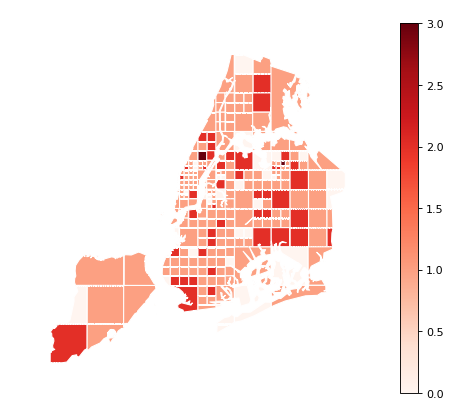# Plot Reference¶

## Pointplot¶

The `pointplot` is a geospatial scatter plot that represents each observation in your dataset as a single point on a map. It is simple and easily interpretable plot, making it an ideal choice for showing simple pointwise relationships between observations.

`pointplot` requires, at a minimum, some points for plotting:

```import geoplot as gplt
import geoplot.crs as gcrs
import geopandas as gpd

gplt.pointplot(cities)
```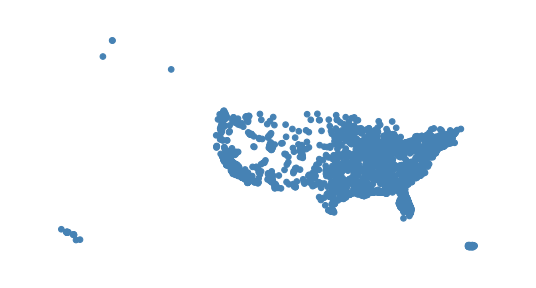The `hue` parameter applies a colormap to a data column. The `legend` parameter toggles a legend.

```gplt.pointplot(cities, projection=gcrs.AlbersEqualArea(), hue='ELEV_IN_FT', legend=True)
```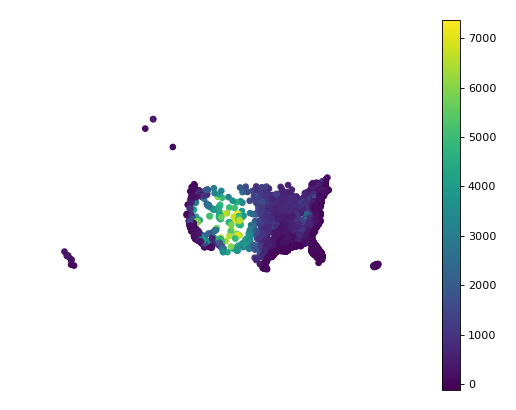Change the colormap using `cmap`. For a categorical colormap, use `scheme`.

```import mapclassify as mc

scheme = mc.Quantiles(cities['ELEV_IN_FT'], k=5)
gplt.pointplot(
cities, projection=gcrs.AlbersEqualArea(),
hue='ELEV_IN_FT', scheme=scheme, cmap='inferno_r',
legend=True
)
```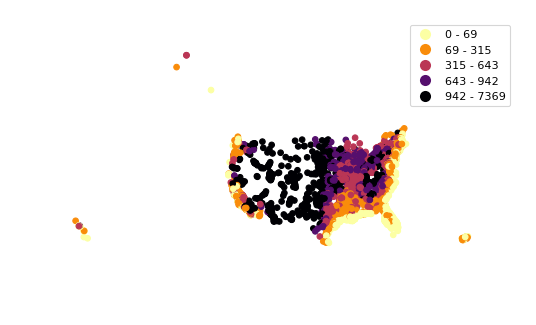Keyword arguments that are not part of the `geoplot` API are passed to the underlying matplotlib.pyplot.scatter instance, which can be used to customize the appearance of the plot. To pass keyword argument to the legend, use the `legend_kwargs` argument.

```gplt.pointplot(
cities, projection=gcrs.AlbersEqualArea(),
hue='ELEV_IN_FT',
legend=True, legend_kwargs={'orientation': 'horizontal'},
edgecolor='lightgray', linewidth=0.5
)
```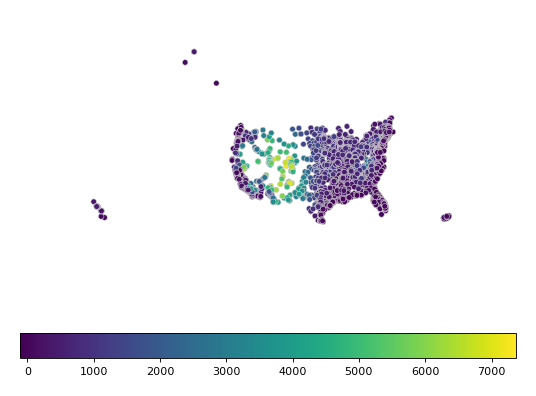`scale` provides an alternative or additional visual variable. The minimum and maximum size of the points can be adjusted to fit your data using the `limits` parameter. It is often benefitial to combine both `scale` and `hue` in a single plot. In this case, you can use the `legend_var` variable to control which visual variable the legend is keyed on.

```gplt.pointplot(
cities, projection=gcrs.AlbersEqualArea(),
hue='ELEV_IN_FT', scale='ELEV_IN_FT', limits=(1, 10), cmap='inferno_r',
legend=True, legend_var='scale'
)
```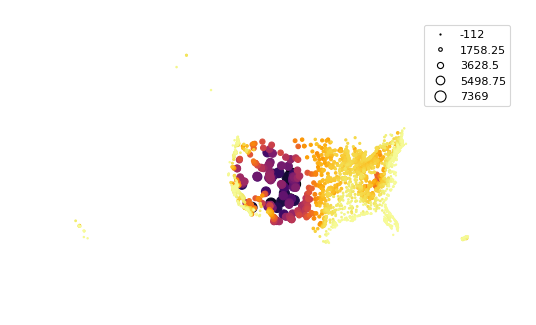## Polyplot¶

The polyplot draws polygons on a map.

```import geoplot as gplt
import geoplot.crs as gcrs
import geopandas as gpd

gplt.polyplot(boroughs, projection=gcrs.AlbersEqualArea())
```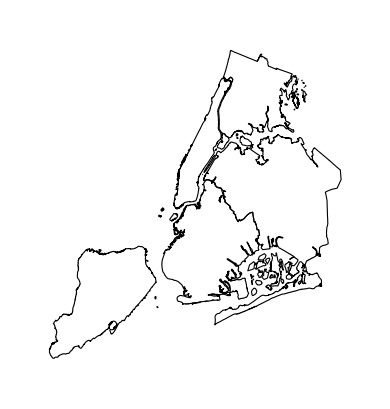`polyplot` is intended to act as a basemap for other, more interesting plot types.

```collisions = gpd.read_file(gplt.datasets.get_path('nyc_collision_factors'))

ax = gplt.polyplot(
boroughs, projection=gcrs.AlbersEqualArea(),
edgecolor='None', facecolor='lightgray'
)
gplt.pointplot(
collisions[collisions['BOROUGH'].notnull()],
hue='BOROUGH', ax=ax, legend=True
)
```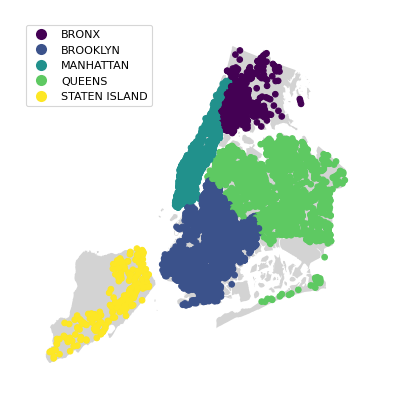## Webmap¶

The webmap creates a static webmap.

```import geoplot as gplt
import geoplot.crs as gcrs
import geopandas as gpd

gplt.webmap(boroughs, projection=gcrs.WebMercator())
```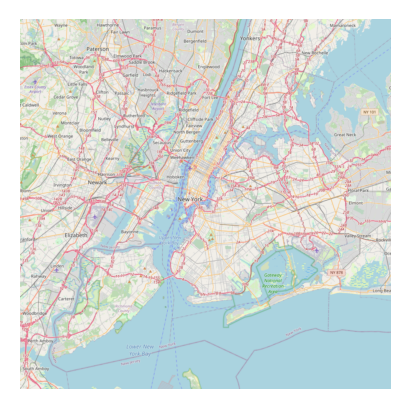`webmap` is intended to act as a basemap for other plot types.

```collisions = gpd.read_file(gplt.datasets.get_path('nyc_collision_factors'))

ax = gplt.webmap(boroughs, projection=gcrs.WebMercator())
gplt.pointplot(
collisions[collisions['BOROUGH'].notnull()],
hue='BOROUGH', ax=ax, legend=True
)
```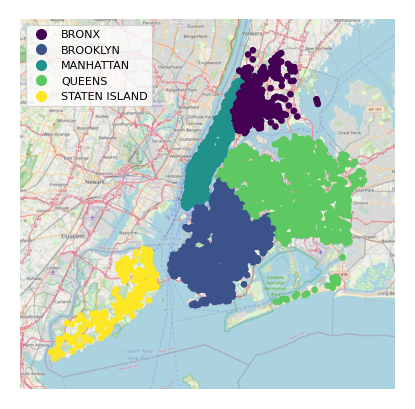## Choropleth¶

A choropleth takes observations that have been aggregated on some meaningful polygonal level (e.g. census tract, state, country, or continent) and displays the data to the reader using color. It is a well-known plot type, and likeliest the most general-purpose and well-known of the specifically spatial plot types.

A basic choropleth requires polygonal geometries and a `hue` variable.

```import geoplot as gplt
import geoplot.crs as gcrs
import geopandas as gpd

gplt.choropleth(contiguous_usa, hue='population')
```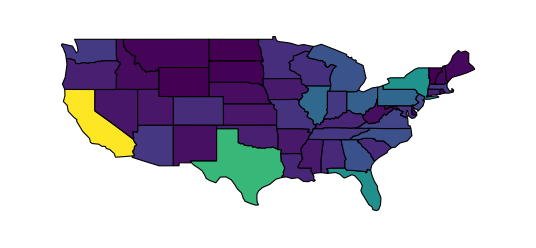Change the colormap using `cmap`. The `legend` parameter toggles the legend.

```gplt.choropleth(
contiguous_usa, hue='population', projection=gcrs.AlbersEqualArea(),
cmap='Greens', legend=True
)
```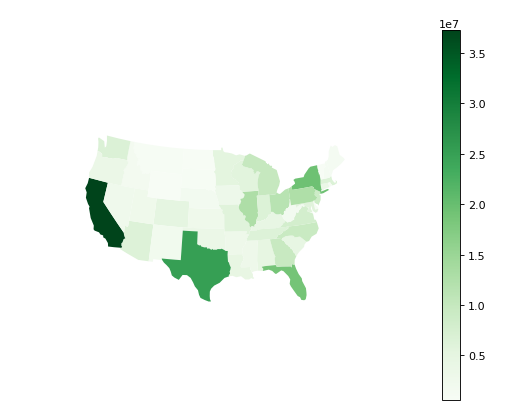Keyword arguments that are not part of the `geoplot` API are passed to the underlying `matplotlib.patches.Polygon` objects; this can be used to control plot aesthetics. To pass keyword argument to the legend, use the `legend_kwargs` argument.

```gplt.choropleth(
contiguous_usa, hue='population', projection=gcrs.AlbersEqualArea(),
edgecolor='white', linewidth=1,
cmap='Greens', legend=True, legend_kwargs={'orientation': 'horizontal'}
)
```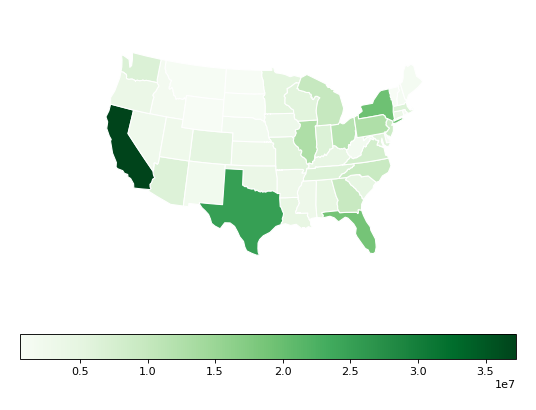To specify a categorical colormap, use `scheme`.

```import mapclassify as mc

scheme = mc.FisherJenks(contiguous_usa['population'], k=5)
gplt.choropleth(
contiguous_usa, hue='population', projection=gcrs.AlbersEqualArea(),
edgecolor='white', linewidth=1,
cmap='Greens',
legend=True, legend_kwargs={'loc': 'lower left'},
scheme=scheme
)
```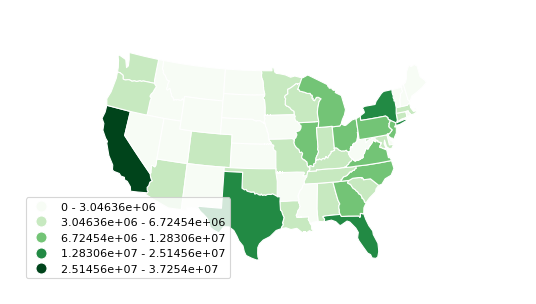Use `legend_labels` and `legend_values` to customize the labels and values that appear in the legend.

```import mapclassify as mc

scheme = mc.FisherJenks(contiguous_usa['population'], k=5)
gplt.choropleth(
contiguous_usa, hue='population', projection=gcrs.AlbersEqualArea(),
edgecolor='white', linewidth=1,
cmap='Greens', legend=True, legend_kwargs={'loc': 'lower left'},
scheme=scheme,
legend_labels=[
'<3 million', '3-6.7 million', '6.7-12.8 million',
'12.8-25 million', '25-37 million'
]
)
```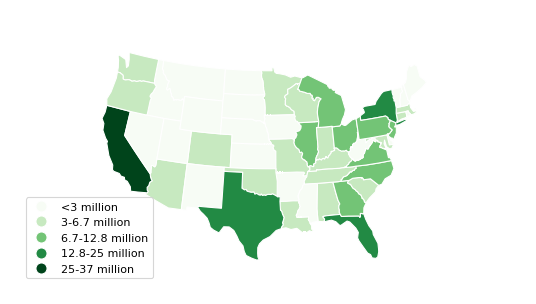## KDEPlot¶

Kernel density estimation is a technique that non-parameterically estimates a distribution function for a sample of point observations. KDEs are a popular tool for analyzing data distributions; this plot applies this technique to the geospatial setting.

A basic `kdeplot` takes pointwise data as input. For interpretability, let’s also plot the underlying borough geometry.

```import geoplot as gplt
import geoplot.crs as gcrs
import geopandas as gpd

ax = gplt.polyplot(boroughs, projection=gcrs.AlbersEqualArea())
gplt.kdeplot(collisions, ax=ax)
```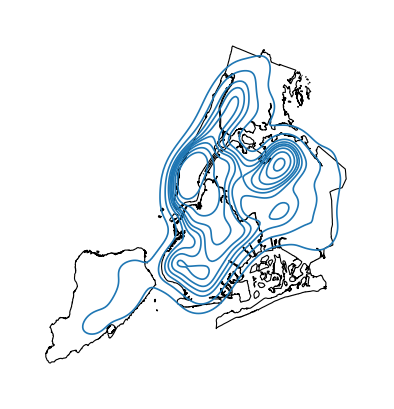`n_levels` controls the number of isochrones. `cmap` control the colormap.

```ax = gplt.polyplot(boroughs, projection=gcrs.AlbersEqualArea())
gplt.kdeplot(collisions, n_levels=20, cmap='Reds', ax=ax)
```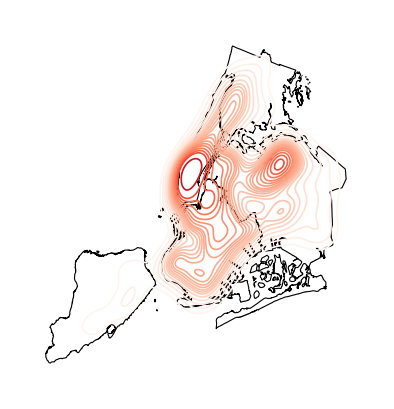`shade` toggles shaded isochrones. Use `clip` to constrain the plot to the surrounding geometry.

```ax = gplt.polyplot(boroughs, projection=gcrs.AlbersEqualArea(), zorder=1)
```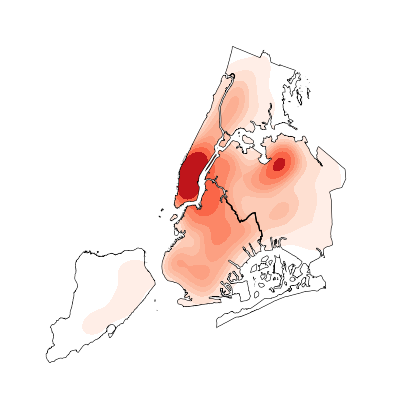Additional keyword arguments that are not part of the `geoplot` API are passed to the underlying seaborn.kdeplot instance. One of the most useful of these parameters is `thresh=0.05`, which toggles shading on the lowest (basal) layer of the kernel density estimate.

```ax = gplt.polyplot(boroughs, projection=gcrs.AlbersEqualArea(), zorder=1)
clip=boroughs, ax=ax)
```## Cartogram¶

A cartogram distorts (grows or shrinks) polygons on a map according to the magnitude of some input data. A basic cartogram specifies data, a projection, and a `scale` parameter.

```import geoplot as gplt
import geoplot.crs as gcrs
import geopandas as gpd

gplt.cartogram(contiguous_usa, scale='population', projection=gcrs.AlbersEqualArea())
```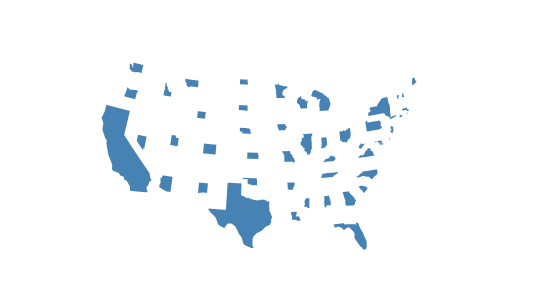Toggle the legend with `legend`. Keyword arguments can be passed to the legend using the `legend_kwargs` argument. These arguments will be passed to the underlying legend.

```gplt.cartogram(
contiguous_usa, scale='population', projection=gcrs.AlbersEqualArea(),
legend=True, legend_kwargs={'loc': 'lower right'}
)
```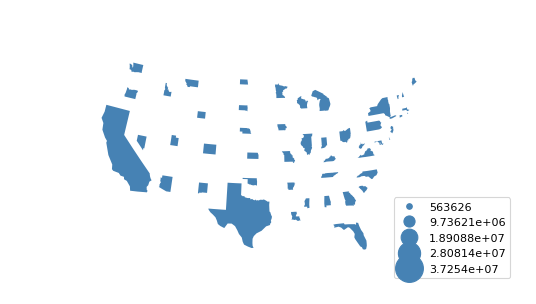To add a colormap to the plot, specify `hue`. Use `cmap` to control the colormap. For a categorical colormap, specify a `scheme`. In this plot we also add a backing outline of the original state shapes, for better geospatial context.

```import mapclassify as mc

scheme = mc.Quantiles(contiguous_usa['population'], k=5)
ax = gplt.cartogram(
contiguous_usa, scale='population', projection=gcrs.AlbersEqualArea(),
legend=True, legend_kwargs={'bbox_to_anchor': (1, 0.9)}, legend_var='hue',
hue='population', scheme=scheme, cmap='Greens'
)
gplt.polyplot(contiguous_usa, facecolor='lightgray', edgecolor='white', ax=ax)
```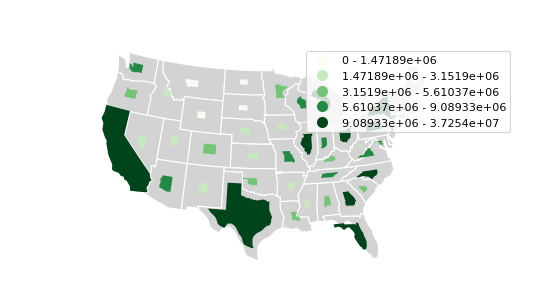Use `legend_labels` and `legend_values` to customize the labels and values that appear in the legend.

```gplt.cartogram(
contiguous_usa, scale='population', projection=gcrs.AlbersEqualArea(),
legend=True, legend_kwargs={'bbox_to_anchor': (1, 0.9)}, legend_var='hue',
hue='population', scheme=scheme, cmap='Greens',
legend_labels=[
'<1.4 million', '1.4-3.2 million', '3.2-5.6 million',
'5.6-9 million', '9-37 million'
]
)
gplt.polyplot(contiguous_usa, facecolor='lightgray', edgecolor='white', ax=ax)
```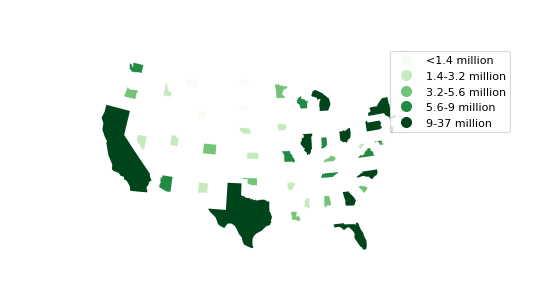Use the `limits` parameter to adjust the minimum and maximum scaling factors. You can also pass a custom scaling function to `scale_func` to apply a different scale to the plot (the default scaling function is linear); see the Pointplot of US city elevations with custom scale functions for an example.

```ax = gplt.cartogram(
contiguous_usa, scale='population', projection=gcrs.AlbersEqualArea(),
legend=True, legend_kwargs={'bbox_to_anchor': (1, 0.9)}, legend_var='hue',
hue='population', scheme=scheme, cmap='Greens',
legend_labels=[
'<1.4 million', '1.4-3.2 million', '3.2-5.6 million',
'5.6-9 million', '9-37 million'
],
limits=(0.5, 1)
)
gplt.polyplot(contiguous_usa, facecolor='lightgray', edgecolor='white', ax=ax)
```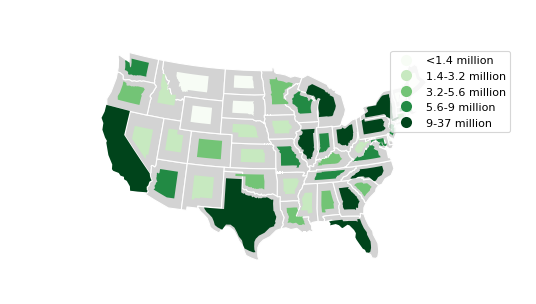## Sankey¶

A Sankey diagram visualizes flow through a network. It can be used to show the magnitudes of data moving through a system. This plot brings the Sankey diagram into the geospatial context; useful for analyzing traffic load a road network, for example, or travel volumes between different airports.

A basic `sankey` requires a `GeoDataFrame` of `LineString` or `MultiPoint` geometries. For interpretability, these examples also include world geometry.

```import geoplot as gplt
import geoplot.crs as gcrs
import geopandas as gpd

ax = gplt.sankey(la_flights, projection=gcrs.Mollweide())
gplt.polyplot(world, ax=ax, facecolor='lightgray', edgecolor='white')
ax.set_global(); ax.outline_patch.set_visible(True)
```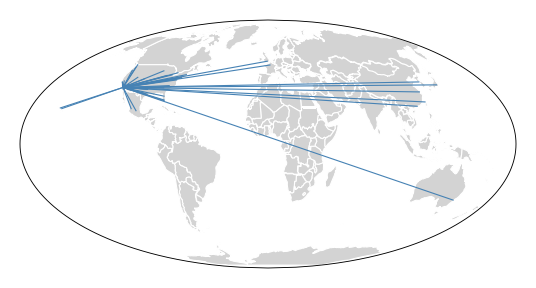`hue` adds color gradation to the map. Use `cmap` to control the colormap. For a categorical colormap, specify `scheme`. `legend` toggles a legend.

```import mapclassify as mc

scheme = mc.Quantiles(la_flights['Passengers'], k=5)
ax = gplt.sankey(
la_flights, projection=gcrs.Mollweide(),
hue='Passengers', cmap='Greens', scheme=scheme, legend=True
)
gplt.polyplot(
world, ax=ax, facecolor='lightgray', edgecolor='white'
)
ax.set_global(); ax.outline_patch.set_visible(True)
```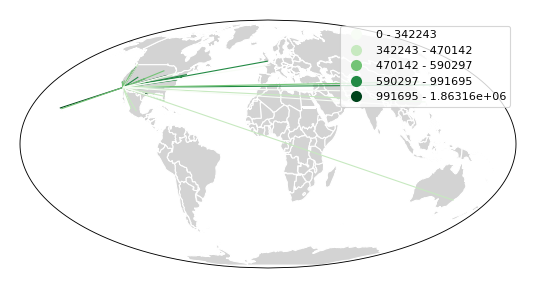`scale` adds volumetric scaling to the plot. `limits` can be used to control the minimum and maximum line width.

```import mapclassify as mc

scheme = mc.Quantiles(la_flights['Passengers'], k=5)
ax = gplt.sankey(
la_flights, projection=gcrs.Mollweide(),
scale='Passengers', limits=(1, 10),
hue='Passengers', cmap='Greens', scheme=scheme, legend=True
)
gplt.polyplot(
world, ax=ax, facecolor='lightgray', edgecolor='white'
)
ax.set_global(); ax.outline_patch.set_visible(True)
```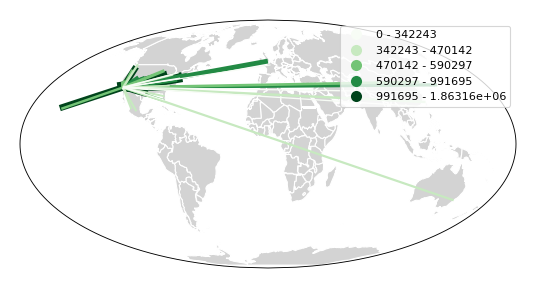Keyword arguments can be passed to the legend using the `legend_kwargs` argument. These arguments will be passed to the underlying legend.

```import mapclassify as mc

scheme = mc.Quantiles(la_flights['Passengers'], k=5)
ax = gplt.sankey(
la_flights, projection=gcrs.Mollweide(),
scale='Passengers', limits=(1, 10),
hue='Passengers', scheme=scheme, cmap='Greens',
legend=True, legend_kwargs={'loc': 'lower left'}
)
gplt.polyplot(
world, ax=ax, facecolor='lightgray', edgecolor='white'
)
ax.set_global(); ax.outline_patch.set_visible(True)
```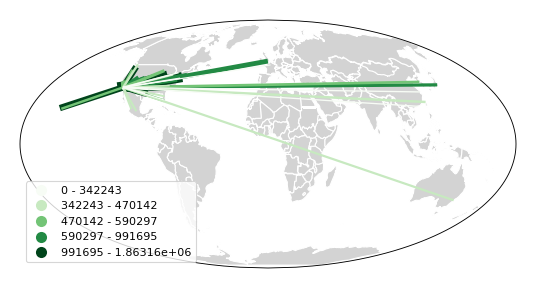## Voronoi¶

The Voronoi region of an point is the set of points which is closer to that point than to any other observation in a dataset. A Voronoi diagram is a space-filling diagram that constructs all of the Voronoi regions of a dataset and plots them.

Voronoi plots are efficient for judging point density and, combined with colormap, can be used to infer regional trends in a set of data.

A basic `voronoi` specifies some point data. We overlay geometry to aid interpretability.

```import geoplot as gplt
import geoplot.crs as gcrs
import geopandas as gpd

gplt.polyplot(boroughs, ax=ax)
```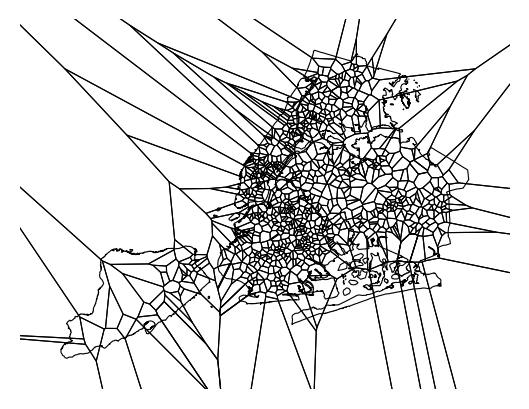Use `clip` to clip the result to surrounding geometry. This is recommended in most cases. Note that if the clip geometry is complicated, this operation will take a long time; consider simplifying complex geometries with `simplify` to speed it up.

```ax = gplt.voronoi(
clip=boroughs.simplify(0.001), projection=gcrs.AlbersEqualArea()
)
gplt.polyplot(boroughs, ax=ax)
```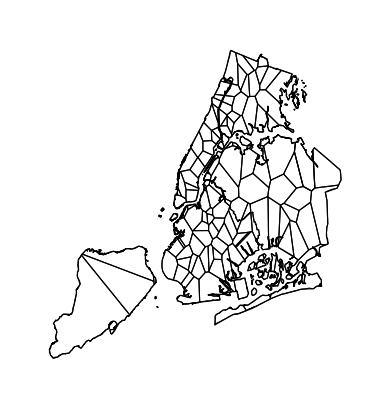Use `hue` to add color as a visual variable to the plot. Change the colormap using `cmap`. To use a categorical colormap, set `scheme`. `legend` toggles the legend.

```import mapclassify as mc

scheme = mc.NaturalBreaks(injurious_collisions['NUMBER OF PERSONS INJURED'], k=3)
ax = gplt.voronoi(
clip=boroughs.simplify(0.001),
hue='NUMBER OF PERSONS INJURED', scheme=scheme, cmap='Reds',
legend=True
)
gplt.polyplot(boroughs, ax=ax)
```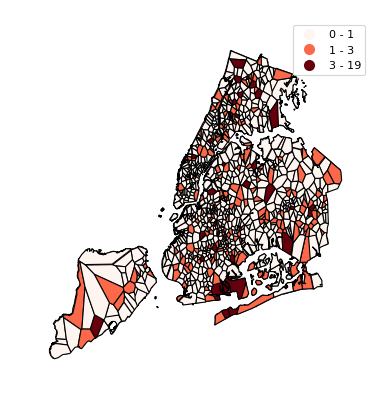Keyword arguments that are not part of the `geoplot` API are passed to the underlying `matplotlib` Polygon patches, which can be used to customize the appearance of the plot. To pass keyword argument to the legend, use the `legend_kwargs` argument.

```import mapclassify as mc

scheme = mc.NaturalBreaks(injurious_collisions['NUMBER OF PERSONS INJURED'], k=3)
ax = gplt.voronoi(
clip=boroughs.simplify(0.001),
hue='NUMBER OF PERSONS INJURED', scheme=scheme, cmap='Reds',
legend=True,
edgecolor='white', legend_kwargs={'loc': 'upper left'}
)
gplt.polyplot(boroughs, edgecolor='black', zorder=1, ax=ax)
```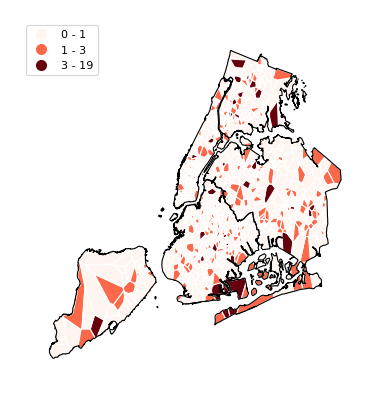A quadtree is a tree data structure which splits a space into increasingly small rectangular fractals. This plot takes a sequence of point or polygonal geometries as input and builds a choropleth out of their centroids, where each region is a fractal quadrangle with at least `nsig` observations.

A quadtree demonstrates density quite effectively. It’s more flexible than a conventional choropleth, and given a sufficiently large number of points can construct a very detailed view of a space.

A simple `quadtree` specifies a dataset. It’s recommended to also set a maximum number of observations per bin, `nmax`. The smaller the `nmax`, the more detailed the plot (the minimum value is 1).

```import geoplot as gplt
import geoplot.crs as gcrs
import geopandas as gpd

```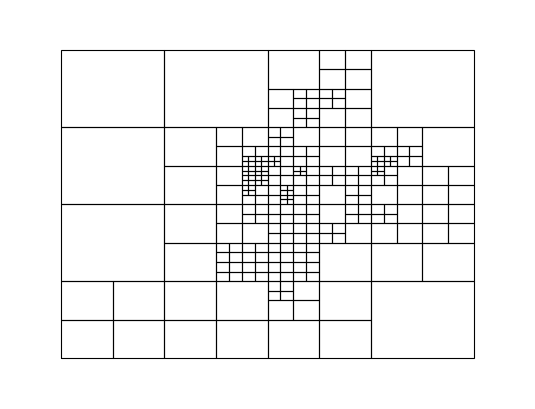Use `clip` to clip the result to surrounding geometry. Note that if the clip geometry is complicated, this operation will take a long time; consider simplifying complex geometries with `simplify` to speed it up.

Keyword arguments that are not part of the `geoplot` API are passed to the underlying matplotlib.patches.Patch instances, which can be used to customize the appearance of the plot.

```gplt.quadtree(
collisions, nmax=1,
projection=gcrs.AlbersEqualArea(), clip=boroughs.simplify(0.001),
facecolor='lightgray', edgecolor='white'
)
```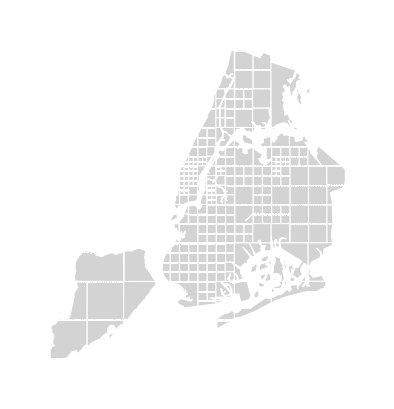A basic clipped quadtree plot such as this can be used as an alternative to `polyplot` as a basemap.

```ax = gplt.quadtree(
collisions, nmax=1,
projection=gcrs.AlbersEqualArea(), clip=boroughs.simplify(0.001),
facecolor='lightgray', edgecolor='white', zorder=0
)
gplt.pointplot(collisions, s=1, ax=ax)
```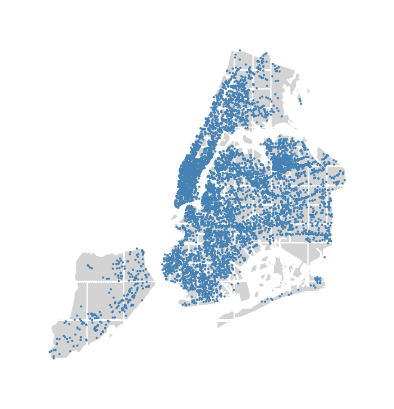Use `hue` to add color as a visual variable to the plot. `cmap` controls the colormap used. `legend` toggles the legend. The individual values of the points included in the partitions are aggregated, and each partition is colormapped based on this aggregate value.

This type of plot is an effective gauge of distribution: the less random the plot output, the more spatially correlated the variable.

The default aggregation function is `np.mean`, but you can configure the aggregation by passing a different function to `agg`.

```gplt.quadtree(
collisions, nmax=1,
projection=gcrs.AlbersEqualArea(), clip=boroughs.simplify(0.001),
hue='NUMBER OF PEDESTRIANS INJURED', cmap='Reds',
edgecolor='white', legend=True,
)
```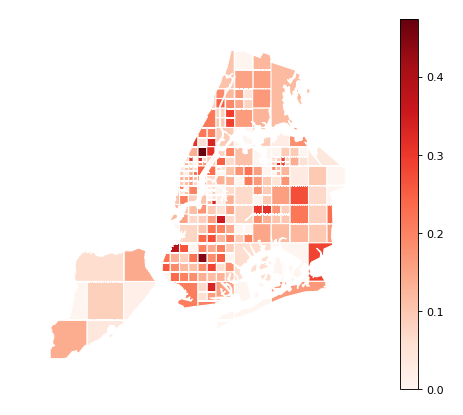To use a categorical colormap, set `scheme`.

```gplt.quadtree(
collisions, nmax=1,
projection=gcrs.AlbersEqualArea(), clip=boroughs.simplify(0.001),
hue='NUMBER OF PEDESTRIANS INJURED', cmap='Reds', scheme='Quantiles',
edgecolor='white', legend=True
)
```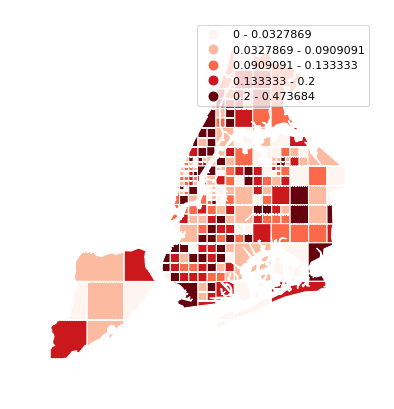Here is a demo of an alternative aggregation function.

```gplt.quadtree(
collisions, nmax=1, agg=np.max,
projection=gcrs.AlbersEqualArea(), clip=boroughs.simplify(0.001),
hue='NUMBER OF PEDESTRIANS INJURED', cmap='Reds',
edgecolor='white', legend=True
)
```